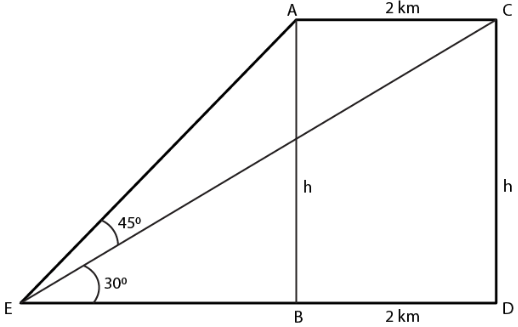Guru

# An aircraft is flying at a constant height with a speed of 360 km/h. From a point on the ground, the angle of elevation of the aircraft at an instant was observed to be 45. After 20 seconds, the angle of elevation was observed to be 30. Determine the height at which the aircraft flying. (use √3 = 1.732)

• 0

sir this is the important  question from the book -ML aggarwal( avichal publication) class 10th , chapter20 , heights and distances
it is given that An aircraft is flying at a constant height with a speed of 360 km/h.
From a point on the ground,
the angle of elevation of the aircraft at an instant was observed to be 450.
After 20 seconds, the angle of elevation was observed to be 300.
Determine the height at which the aircraft flying. (use √3 = 1.732)

question no 38 , heights and distances , ICSE board, ML Aggarwal

Share

1. It is given that

Speed of aircraft = 360 km/h

Distance covered by the aircraft in 20 seconds = (360 × 20)/ (60 × 60) = 2 km

Consider E as the fixed point on the ground

CD as the position of AB in height of aircraftTake AB = CD = h km

In right triangle ARB

tan θ = AB/ EB

Substituting the values

tan 450 = h/EB

1 = h/EB

EB = h

Here

ED = EB + BD = h + 2 km

In right triangle CED

tan 300 = CD/ED

Substituting the values

1/√3 = h/ (h + 2)

√3h = h + 2

1.732h – h = 2

0.732h = 2

We know that 2km = 2000 m

h = 2000/0.732

h = (2000 × 1000)/ 732 = 2732 m

• 1